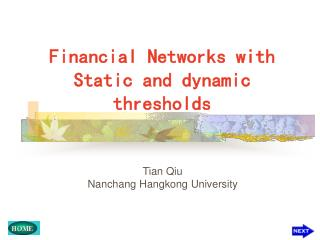DownloadDownload PresentationFinancial Networks with Static and dynamic thresholds

# Financial Networks with Static and dynamic thresholds

Télécharger la présentation## Financial Networks with Static and dynamic thresholds

- - - - - - - - - - - - - - - - - - - - - - - - - - - E N D - - - - - - - - - - - - - - - - - - - - - - - - - - -
##### Presentation Transcript

1. Financial Networks with Static and dynamic thresholds Tian Qiu Nanchang Hangkong University

2. Outline • Motivation • Financial networks with static and dynamic thresholds • Topology dynamics • Economic sectors • Conclusions

3. Motivation We introduce a dynamic financial network with both static and dynamic thresholds based on the daily data of the American and Chinese stock markets, and investigate the topology dynamics, such as the average clustering coefficient, the average degree and the cross correlation of degrees. Special attention is focused on dynamic effect of the thresholds on the network structure and network stability.

4. Financial networks with static and dynamic thresholds

5. Financial networks with static and dynamic thresholds We define the price return We normalize the price return to where

6. Financial networks with static and dynamic thresholds We define an instantaneous equal-time cross-correlation between two stocks by take individual stocks as nodes and set a threshold to create links. At each time step, if the cross correlation , then add a link between stocks i and j ; otherwise, cut the link.

7. Financial networks with static and dynamic thresholds static threshold

8. Financial networks with static and dynamic thresholds dynamic threshold

9. Topology dynamics • detrended fluctuation analysis(DFA) • Average clustering coefficient • Average degree • cross correlation of degrees

10. Topology dynamics-detrended fluctuation analysis(DFA) For a time series A(t’’), we eliminate the average trend from the time series by introducing Uniformly dividing [1, T ] into windows of size t and fitting B(t’) to a linear function in each window, we define the DFA function as

11. Topology dynamics-detrended fluctuation analysis(DFA) In general, F(t) will obey a power-law scaling behavior indicate anti-correlated time series indicate the Gaussian white noise indicate long-range correlating time series indicate noise indicate unstable time series

12. Topology dynamics-Average clustering coefficient where is the clustering coefficient of node The average clustering coefficient is defined by

13. Topology dynamics-Average clustering coefficient 0.88 0.78 0.68 0.85

14. Topology dynamics-Average clustering coefficient

15. Topology dynamics-Average degree where is the degree of node The average degree is defined by

16. Topology dynamics-Average degree

17. Topology dynamics-Average degree

18. Why is the dynamic threshold crucial? One important reason is that the volatilities fluctuate strongly in the dynamic evolution, especially on the crash days. It induces large temporal fluctuations of the cross correlations of price returns. Thus the static threshold leads to dramatic changes in the topological structure of the network. However, the dynamic threshold proportional to suppresses such kinds of fluctuations and results in a stable topological structure of the network.

19. Why is the dynamic threshold crucial?

20. Degree distribution

21. Topology dynamics-cross correlation of degrees The so-called assortative or disassortative mixing on the degrees refers to the cross correlation of degrees. ‘Assortative mixing’ means that high-degree nodes tend to directly connect with high-degree nodes, while ‘disassortative mixing’ indicates that high-degree nodes prefer to directly connect with low-degree nodes.

22. Topology dynamics-cross correlation of degrees where and are the degrees of the nodes at both ends of the link, with The cross correlation of degrees is defined as represent assortative mixing, no assortative mixing and disassortative mixing, respectively.

23. Topology dynamics-cross correlation of degrees 0.00 0.36 -0.20 0.22

24. Topology dynamics-cross correlation of degrees

25. Economic sectors

26. Topology dynamics- Economic sectors we first introduce the normalized individual degrees We then construct the cross correlation matrix F of individual degreeswhose elements are and compute its eigenvalues and eigenvectors.

27. Topology dynamics- Economic sectors A:basic materials; B: conglomerates; C: consumer goods; D: finance; E: healthcare;F: industrial goods; G: services; H: technology; I: utilities.

28. Topology dynamics- Economic sectors

29. Conclusions • the dynamic threshold properly suppresses the large fluctuation induced by the cross correlations of individual stock prices and creates a rather robust and stable network structure during the dynamic evolution, in comparison to the static threshold. • Long-range time correlations are revealed for the average clustering coefficient, the average degree and the cross correlation of degrees.

30. Thank You!